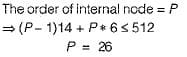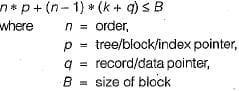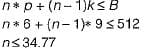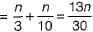# Test: File Structures- 2

## 12 Questions MCQ Test GATE Computer Science Engineering(CSE) 2023 Mock Test Series | Test: File Structures- 2

Description
Attempt Test: File Structures- 2 | 12 questions in 35 minutes | Mock test for Computer Science Engineering (CSE) preparation | Free important questions MCQ to study GATE Computer Science Engineering(CSE) 2023 Mock Test Series for Computer Science Engineering (CSE) Exam | Download free PDF with solutions
QUESTION: 1

Solution:
QUESTION: 2

### Which allocation scheme would work best for a file system implemented on a device that can only be accessed sequentially, a tape drive, for instance?

Solution:

Contiguous allocation scheme would work best for a file system implementation on a device that can only be accessed sequentially, a tape drive.

QUESTION: 3

### Consider the following statements: S1 : Anchor block contains first records of chain. S2:  Anchor record is first record of a block. Which of the above statements is/are true?

Solution:
• Anchor block contains first records of chain, while overflow block contains records other than those that are 1st records of a chain.
• Anchor record is first record of a block.
QUESTION: 4

Which statement is false?

Solution:
QUESTION: 5

Which indices search key defines the sequential order of file and which indices search key specifies an order different from sequential order of file?

Solution:

Primary indices search key defines the sequential order of fill while secondary indices search key defines an order different from sequential order of fills.

QUESTION: 6

Which of the following is correct?

Solution:

Most database systems use indexes built on some form of a B+ tree due to its many advantages, in particular its support for range queries. Leaf nodes are linked together in B* trees hence range queries are faster.

QUESTION: 7

The order of an internal node in a B+ tree index is the maximum number of children it can have. Suppose that a child pointer takes 6 bytes, the search field value takes 14 bytes, and the block size is 512 bytes. What is the order of the internal node?

Solution:

Size of child pointer = 6 byte
Size of search field value takes 14 bytes Block size = 512QUESTION: 8

A B tree used as an index for a large database table has four levels including the root node. If a new key is inserted in this index, then the maximum number of nodes that could be newly created in the process are

Solution:

Suppose all nodes are completely full that means every node has n - 1 keys.
Tree has 4 levels. If a new key is inserted then at every level new node will be created.
In worst case root node will also be broken into two parts and we have 4 levels.
So answer should be 5 because tree will be increased with one more level.

QUESTION: 9

In a database file structure, the search key field is 9 bytes long, the block size is 512 bytes, a record pointer is 7 bytes and a block pointer is 6 bytes. The largest possible order of a leaf node in a B+ tree implementing this file structure is

Solution:

From the structure of B+ tree we can get the following equation:In B+ tree, non leaf node has no record pointer: (by putting q = 0)Largest possible order of a non-leaf node = 34.

QUESTION: 10

With respect to the B+ tree index method, select the true statements

Solution:

In B+ tree index method records are physically stored in primary key order.

QUESTION: 11

Given a block can hold either 3 records or 10 key pointers. A database contains n records, then how many blocks do we need to hold the data file and the dense index.

Solution:

For storing the records no. of blocks required =n/3
and For storing the keys in dense index no. of blocks required =n/10
So total blocks requiredQUESTION: 12

Which is the best suitable for sequential access of data?

Solution:

B+ tree is best suitable for sequential acess of data.Use Code STAYHOME200 and get INR 200 additional OFF Use Coupon Code